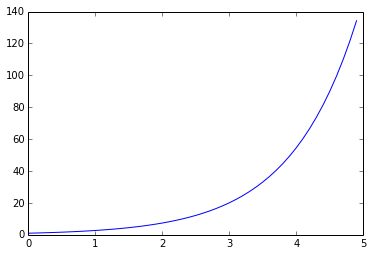1. # Analysis of Shakespeare character speech topics

In Part I of this post I made a topic model of the speech of Shakespeare characters from eight plays. Here in Part II I'll analyze the results of the model. Download notebook.

# Introduction¶

I recently starting collecting data from the BART API, specifically estimated time to departure for trains at the two stations I use most frequently. In this notebook I'll show how I parsed the data from a csv file, reshaped it to fit the questions at hand, and made a few plots. Download notebook ...read more

3. # Label graph axes!

It's easy enough to make a plot using matplotlib.

```import matplotlib.pyplot as plt
import numpy as np

time_point_array = np.arange(0, 5, .1)
y_value_array = np.exp(time_point_array)

plt.plot(time_point_array, y_value_array)
```This plot is not great data science. In fact it's poor data …